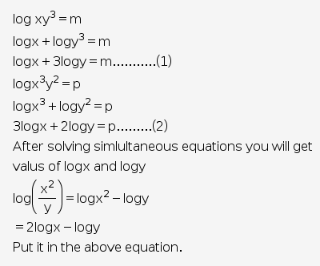# If log xy^3=m and log x^3 y^2=p,find log (x^2 ÷ y) in term of m and p

If log xy3=m and log x3 y2=p,find log (x2 ÷ y) in term of m and p